# IT Blog

Modelling & Forecasting Time-Series data has been one of the cornerstones of Predictive Analytics in the era of Big Data.Modelling & Forecasting Time-Series data has been one of the cornerstones of Predictive Analytics in the era of Big Data. There are a plethora of forecasting techniques available today whose context can be a pain to understand and as we know, in the war on noise, context serves as a crucial ammunition. To that end, we, from VitalClick are presenting this series of articles where we will be discussing a structured methodology to understand, analyse & forecast time-series data

In the previous article, we have explored the Exponential Smoothing framework for time-series forecasting. ARIMA models are built on a different yet complementary approach. ARIMA models have many variants among themselves tailored for a wide range of time-series exhibiting different structural characteristics such as Seasonal ARIMA models, ARIMA with External Regressors, Fractional ARIMA etc. However, to understand these specific case-extensions of ARIMA, it is imperative to understand the subtle specificities in the Mathematical theory behind ARIMA models. With our aim being to gain a practical understanding of the concepts behind time-series modelling, we will defer a deep-dive analysis of the mathematical structure of ARIMA modelling to a later period (maybe a different series) and we will take our first step towards understanding the ARIMA framework.

#### CONCEPT OF AUTO-REGRESSION

Similar to Exponential Smoothing models, AR models involve interpreting a particular observation of the time-series as a function of its preceding observations. However, in Exponential Smoothing we expressed an observation as the weighted average of its preceding values with exponentially decreasing weights, where as in our current scenario we express a particular data-point as a regression of the preceding observations., hence the name Auto-Regression (which means Self- Regression).

Let us look at a generic multiple regression model: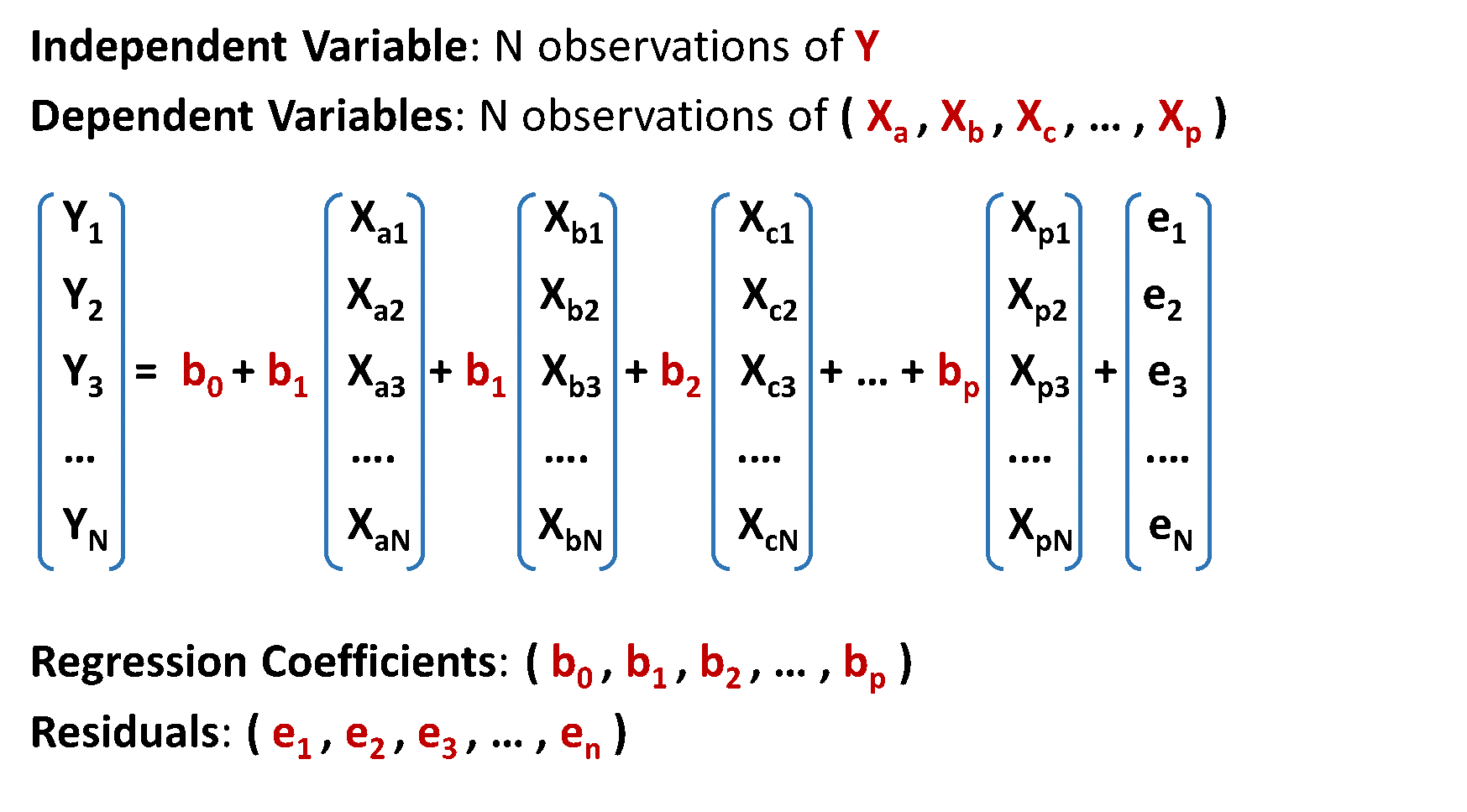To make valid statistical inferences from the above model, we make the following assumptions;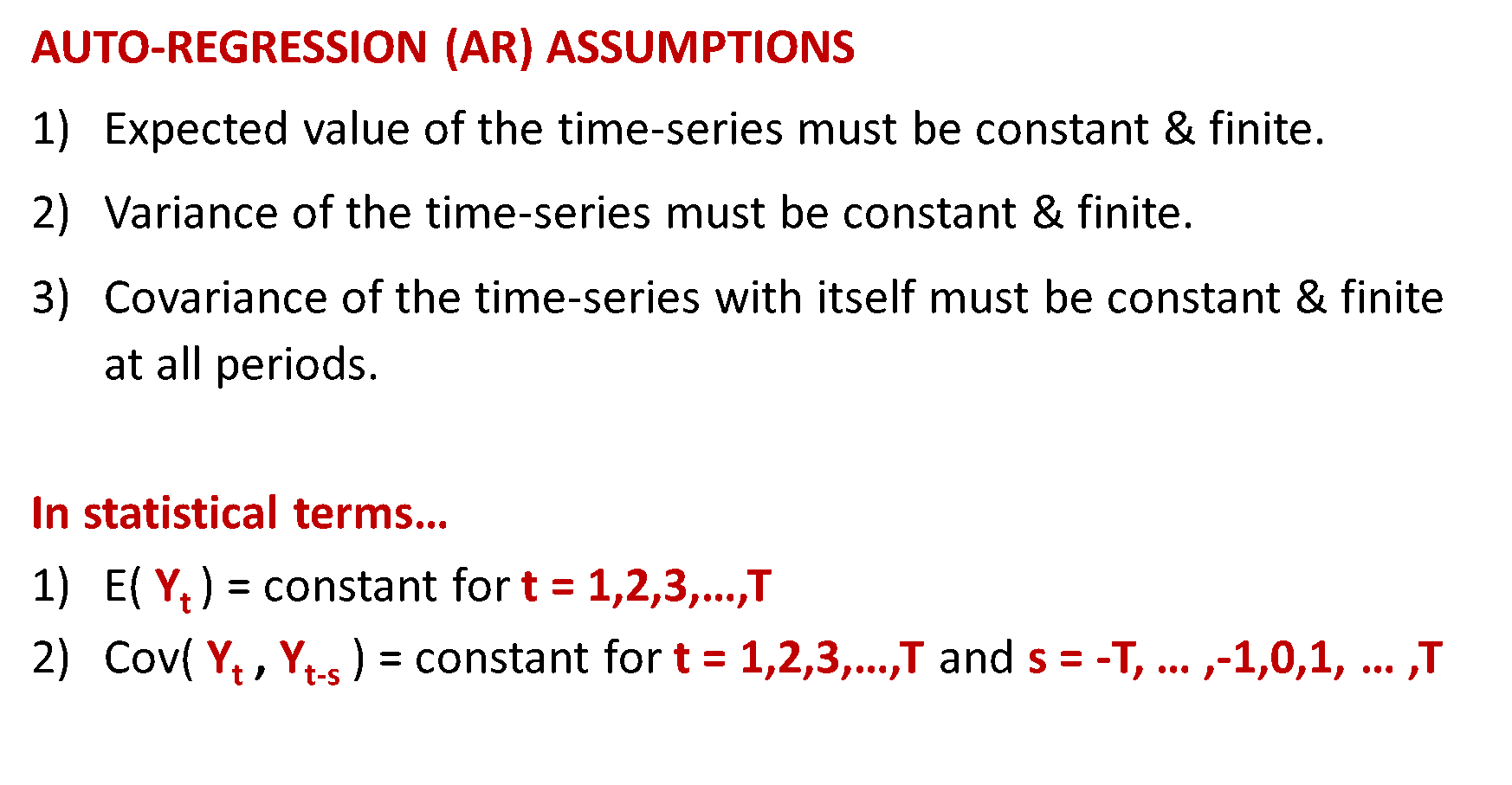The same conditions hold for the validity of an Auto-Regression, in case of a time-series. Let us consider an Auto-Regressive (AR) model of order p as shown:Translating the above conditions to the context of a time-series results in the following assumptions;These set of pre-requisites for the validity of an application of an AR Model are collectively called as the condition of Covariance Stationarity

#### CONCEPT OF DIFFERENCING

Let us understand the pre-requisite condition of Covariance Stationarity for AR models using a simple generic example of a Random Walk time-series.A Random Walk is a time-series in which the value of the series at a particular time is equal to its value in the previous period plus an error that has constant variance and is uncorrelated with the error term in the previous periods. We can mathematically express it in the following way.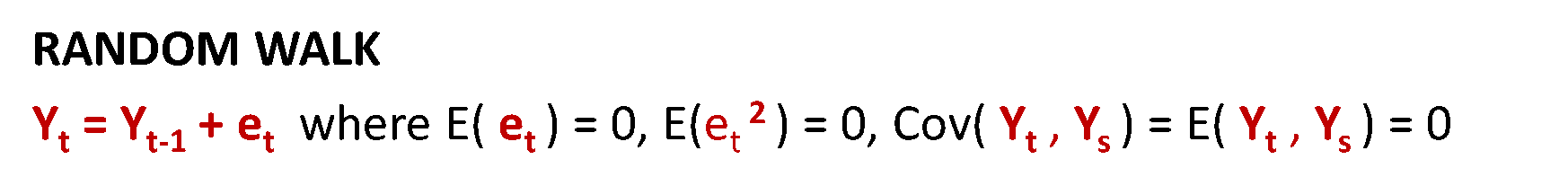Looking at the above mathematical formulation of a Random Walk series, can it be represented by an Auto-Regressive model? Let us examine.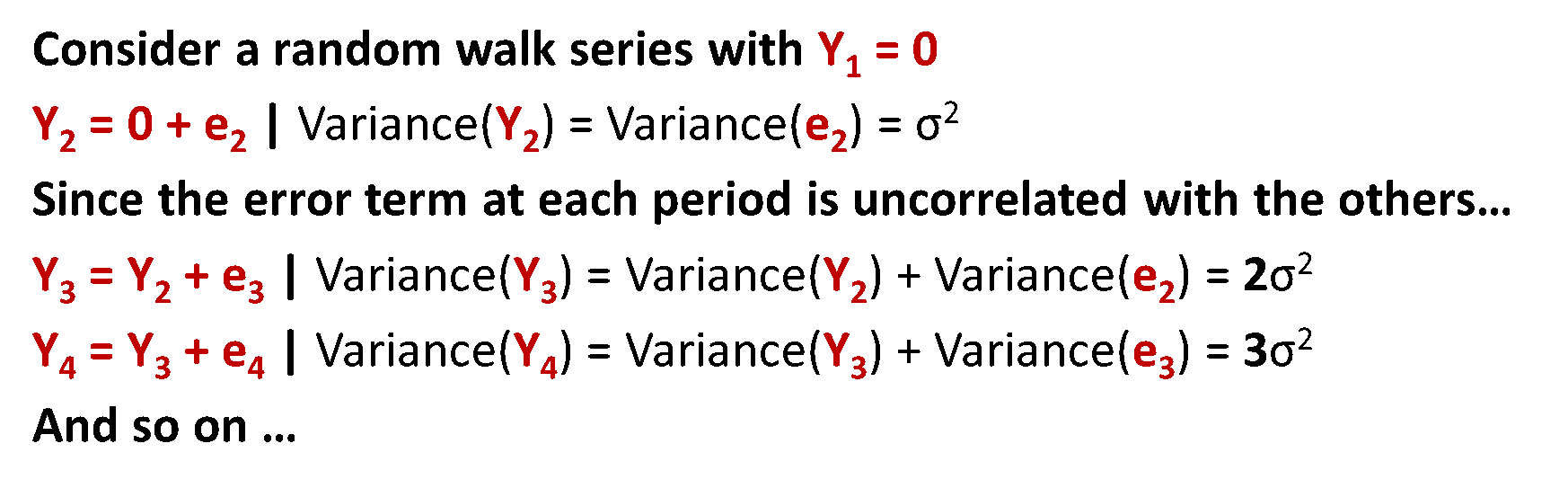Since the random walk series doesn’t comply with the Covariance Stationarity condition of constant variance, we can’t use Auto-Regression Analysis on the Random Walk series. We can however attempt to convert the time-series to a stationary series using mathematical transformations.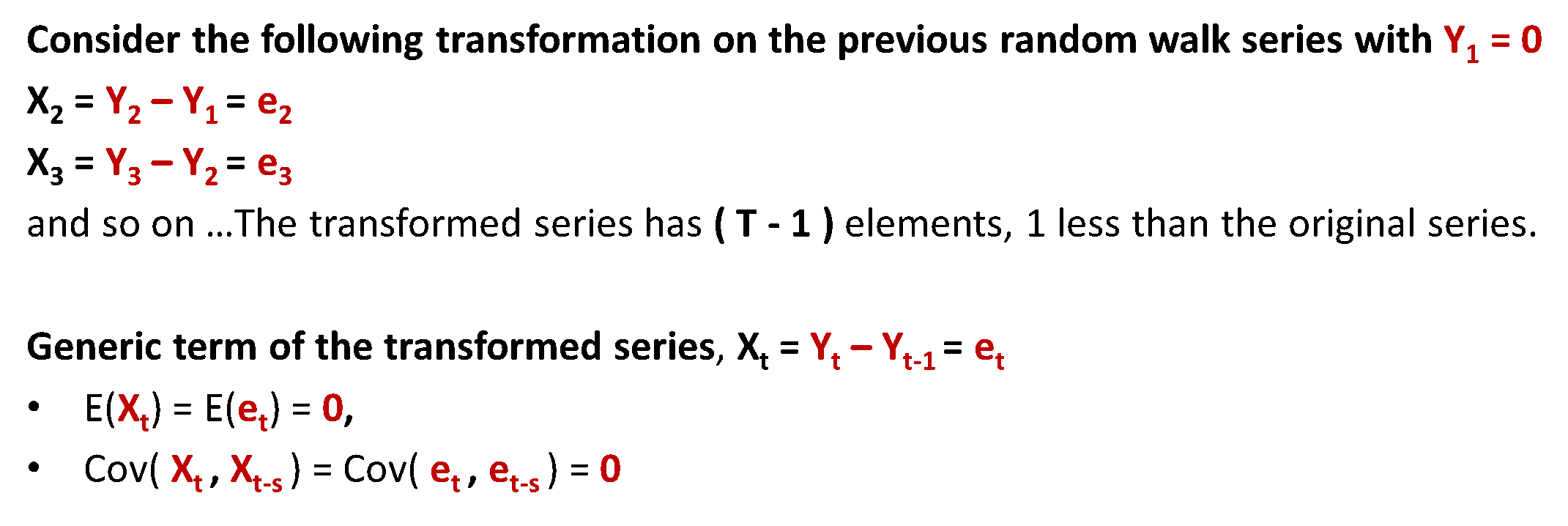As we have seen in the above example of a Random Walk series and as we can prove for any other series, differencing is one of the transformations we apply on time-series so as to make it comply to the Covariance Stationarity principle. Differencing not only makes a series mean-reverting (condition 1 of the Covariance Stationarity principle), it also voids any inherent correlation between consecutive entities of the time-series (condition 2 of the Covariance Stationarity principle).

There are two attributes to the Differencing Transformation;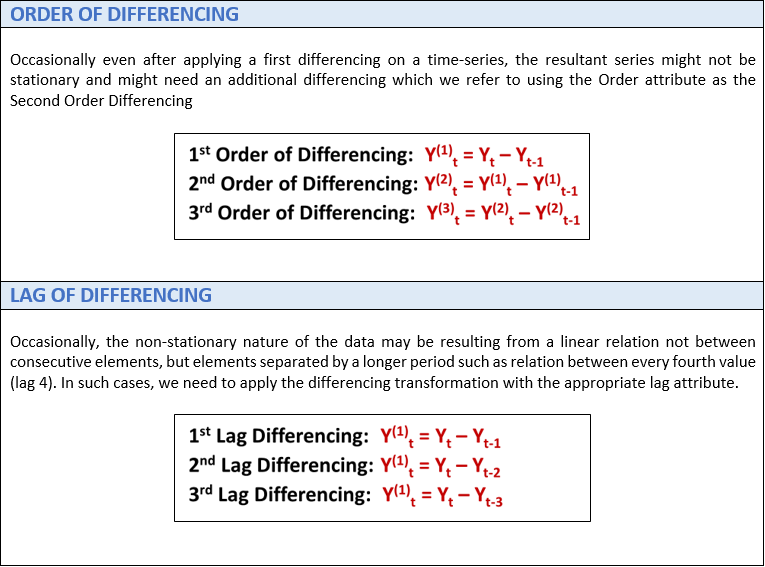MOVING AVERAGE MODELS

Moving Average (MA) models though contextually similar, differ from the Auto-Regression (AR) models in the way that MA models interpret a time-series observation as a regression of the past forecast errors rather than the past values of the variable. A Moving Average model of order q can be mathematically formulated as shown;br/>>Since the regressor variables in the Moving Average model are the uncorrelated errors, the Covariance Stationarity principle isn’t relevant.
Moving Average (MA) models should not be confused with Moving Average (MA) smoothing. A moving average model is used for forecasting future values while moving average smoothing is used for estimating the trend-cycle of past values.

AUTO-REGRESSIVE (AR) INTEGRATED (I) MOVING AVERAGE (MA) MODELS (ARIMA)

So far we have considered Auto-Regressive (AR) & Moving Average (MA) models as alternatives for modelling a time-series. We can combine the p auto-regressive lags of the variable with an order of differencing d and the q moving average terms to form a generalised model, which can be denoted by ARIMA(p,d,q) as shown;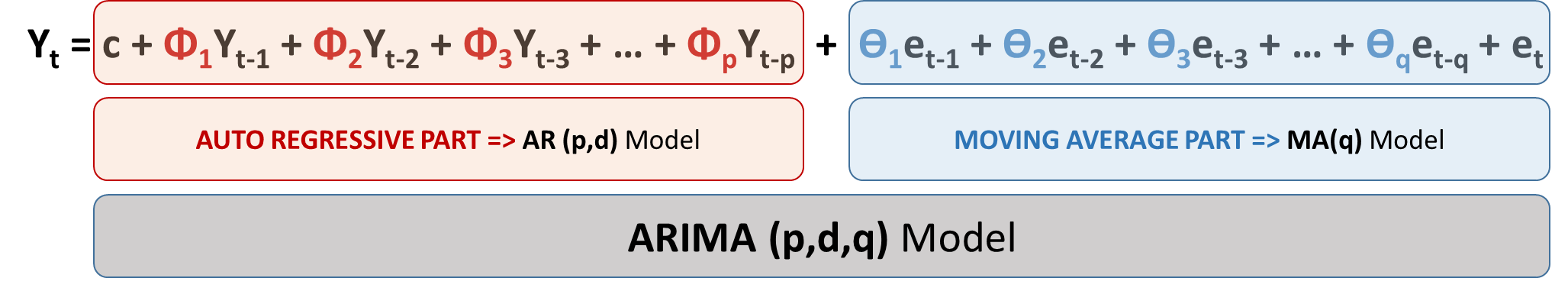``````

IMPLEMENTATION IN R

require(datasets)
require(forecast)

# EuStockMarkets is an inherent time-series object in R containing the daily closing...
# prices of 4 major european stock indices, of which we are considering the UK FTSE.
data <- EuStockMarkets[,4]
plot(data)

model <- auto.arima( x = data, max.p = 5, max.q = 5, max.d = 2 )
print(model)
# auto.arima() returns the best ARIMA model based on a criterion of the user's...
# choice (AIC, AICc, BIC) by performing a grid search on ARIMA models with different...
# values of p, d & q.

?auto.arima
# auto.arima() needs a time-series object as a mandatory input.
# In addition, it provides us with arguments for the constraints on the grid-search such as
# ---> max.p - Maximum value of p to be tested (default value = 5)
# ---> max.q - Maximum value of q to be tested (default value = 5)
# ---> max.d - Maximum value of d to be tested (default value obtained from KPSS test)
# among the numerous other arguments.

frcst <- forecast(object = model, h = 20)
plot(frcst)
``````
```OUTPUT

```

#### EXTENSIONS OF ARIMA MODELS

Unlike most of the time-series models, the foundations of the ARIMA framework can be traced to the subject of Econometrics for modelling economic time-series. Computing the parameters of an ARIMA model, though conceptually straight-forward, needs an elaborate procedure to understand which needs a much deeper insight into the structural properties of time-series like Stationarity, Stochastic vs Deterministic series etc.

These are beyond the scope of any single presentation and particularly our current series where we aim to strike a balance between understanding the mathematical complexities & gaining a practical outlook on time-series modelling . But rest assured, we are not abandoning the study on ARIMA framework. Instead, we will be deferring our study to a different series where we will build on our current understanding and deep-dive into the mathematical subtleties behind the ARIMA models.

Keep watching this space for more.